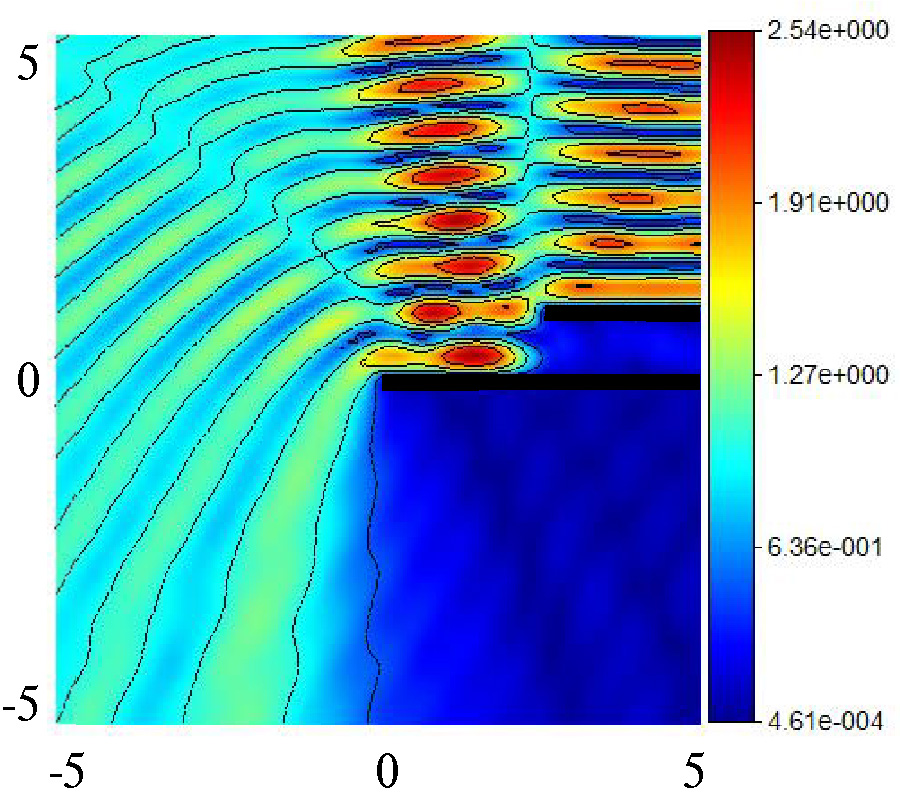# Vol. 101

Front:[PDF file] Back:[PDF file]
Latest Volume
All Volumes
All Issues
2021-03-20

#### Diffraction of the Electromagnetic Plane Waves by Double Half-Plane with Fractional Boundary Conditions

By Vasil Tabatadze, Kamil Karaçuha, Eldar I. Veliyev, and Ertuğrul Karaçuha
Progress In Electromagnetics Research M, Vol. 101, 207-218, 2021
doi:10.2528/PIERM21012003

## Abstract

In this article, the diffraction of E-polarized electromagnetic plane waves by a double half-plane structure is taken into account. The shift of the upper half-plane through the horizontal axis for different wavenumber and boundary conditions are considered. On the double half-plane structure, fractional boundary conditions are required on the half-plane surfaces. The half-planes are parallel to each other with a variable shift in distance and location. The formulation of the problem is given where the boundary condition is explained, and the integral equations for each half-plane are obtained by using fractional calculus and Fourier Transform techniques. Then, for numerical calculations, the induced current on each half-plane is expressed as the summation of Laguerre polynomials. This leads to having a system of linear algebraic equations needed to be solved. The numerical results show that the shift and the distance between the half-planes give a very important effect on the field values inside and outside the guiding structure. The results are compared and analyzed with Method of Moment and previous results.

## Citation

Vasil Tabatadze, Kamil Karaçuha, Eldar I. Veliyev, and Ertuğrul Karaçuha, "Diffraction of the Electromagnetic Plane Waves by Double Half-Plane with Fractional Boundary Conditions," Progress In Electromagnetics Research M, Vol. 101, 207-218, 2021.
doi:10.2528/PIERM21012003
http://www.jpier.org/PIERM/pier.php?paper=21012003

## References

1. Mittra, R. and S. W. Lee, Analytical Technique in the Theory of Guided Waves, The Macmillan Company, 1971.

2. Nethercote, M. A., R. C. Assier, and I. D. Abrahams, "Analytical methods for perfect wedge diffraction: A review," Wave Motion, Vol. 93, 102479, 2020.
doi:10.1016/j.wavemoti.2019.102479

3. Rudduck, R. and L. Tsai, "Aperture reflection coefficient of TEM and TE01 mode parallel-plate waveguides," IEEE Transactions on Antennas and Propagation, Vol. 16, No. 1, 83-89, 1968.
doi:10.1109/TAP.1968.1139123

4. Hame, Y. and I. H. Tayyar, "Plane wave diffraction by dielectric loaded thick-walled parallel-plate impedance waveguide," Progress In Electromagnetics Research, Vol. 44, 143-167, 2004.

5. Zheng, J.-P. and K. Kobayashi, "Plane wave diffraction by a finite parallel-plate waveguide with four-layer material loading: Part 1 - The case of E-polarization," Progress In Electromagnetics Research B, Vol. 6, 1-36, 2008.
doi:10.2528/PIERB08031219

6. Alkumru, A., "Plane wave diffraction by three parallel thick impedance half-planes," Journal of Electromagnetic Waves and Applications, Vol. 12, No. 6, 801-819, 1998.
doi:10.1163/156939398X01051

7. Tiberio, R. and R. Kouyoumjian, "Calculation of the high-frequency diffraction by two nearby edges illuminated at grazing incidence," IEEE Transactions on Antennas and Propagation, Vol. 32, No. 11, 1186-1196, 1984.
doi:10.1109/TAP.1984.1143244

8. Umul, Y. Z., "Diffraction of waves by a boundary between two half-planes with different resistivities," Optics Letters, Vol. 40, No. 7, 1306-1309, 2015.
doi:10.1364/OL.40.001306

9. Umul, Y. Z., "Wave diffraction by a reflectionless half-plane," Applied Optics, Vol. 56, No. 33, 9293-9300, 2017.
doi:10.1364/AO.56.009293

10. Basdemir, H. D., "Scattering of plane waves by a rational half-plane between DNG media," Optik, Vol. 179, 47-53, 2019.
doi:10.1016/j.ijleo.2018.10.113

11. Veliyev, E. I., V. Tabatadze, K. Karaçuha, and E. Karaçuha, "The diffraction by the half-plane with the Fractional boundary condition," Progress In Electromagnetics Research M, Vol. 88, 101-110, 2020.
doi:10.2528/PIERM19102408

12. Tabatadze, V., K. Karaçuha, E. I. Veliyev, and E. Karaçuha, "The diffraction by two half-planes and wedge with the fractional boundary condition," Progress In Electromagnetics Research M, Vol. 91, 1-10, 2020.
doi:10.2528/PIERM20020503

13. Veliev, E. I., T. Ahmedov, and M. Ivakhnychenko, Fractional Operators Approach and Fractional Boundary Conditions, Electromagnetic Waves, (ed.) Vitaliy Zhurbenko, IntechOpen, 2011, doi: 10.5772/16300.

14. Engheta, N., "Use of fractional integration to propose some ``fractional'' solutions for the scalar Helmholtz equation," Progress In Electromagnetics Research, Vol. 12, 107-132, 1996.

15. Engheta, N., "Fractional curl operator in electromagnetics," Microwave and Optical Technology Letters, Vol. 17, No. 2, 86-91, 1998.
doi:10.1002/(SICI)1098-2760(19980205)17:2<86::AID-MOP4>3.0.CO;2-E

16. Engheta, N., "On fractional calculus and fractional multipoles in electromagnetism," IEEE Transactions on Antennas and Propagation, Vol. 44, No. 4, 554-566, 1996.
doi:10.1109/8.489308

17. Veliev, E. I. and N. Engheta, "Generalization of Green's theorem with fractional differintegration," Proceedings of IEEE AP-S International Symposium & USNC/URSI National Radio Science Meeting, 2003.

18. Engheta, N., "Fractionalization methods and their applications to radiation and scattering problems," Proceedings of International Conference on Mathematical Methods in Electromagnetic Theory, 34-40, 2000.

19. Ivakhnychenko, M., E. Veliev, and T. Ahmedov, "Scattering properties of the strip with fractional boundary conditions and comparison with the impedance strip," Progress In Electromagnetics Research C, Vol. 2, 189-205, 2008.
doi:10.2528/PIERC08031502

20. Karaçuha, K., E. I. Veliyev, V. Tabatadze, and E. Karaçuha, "Application of the method of fractional derivatives to the solution of the problem of plane wave diffraction by two axisymmetric strips of different sizes," Proceedings of URSI International Symposium on Electromagnetic Theory (EMTS), San Diego, USA, 2019.

21. Tabatadze, V., K. Karaçuha, and E. I. Veliev, "The fractional derivative approach for the diffraction problems: Plane wave diffraction by two strips with the fractional boundary conditions," Progress In Electromagnetics Research C, Vol. 95, 251-264, 2019.
doi:10.2528/PIERC19062505

22. Karaçuha, K., E. I. Veliyev, V. Tabatadze, and E. Karaçuha, "Analysis of current distributions and radar cross sections of line source scattering from impedance strip by fractional derivative method," Advanced Electromagnetics, Vol. 8, No. 2, 108-113, 2019.
doi:10.7716/aem.v8i2.981

23. Podlubny, I., Fractional Differential Equations: An Introduction to Fractional Derivatives, Fractional Differential Equations, to Methods of Their Solution and Some of Their Applications, Elsevier, 1998.

24. Prudnikov, H. P., Y. H. Brychkov, and O. I. Marichev, Special Functions, Integrals and Series, Vol. 2, Gordon and Breach Science Publishers, 1986.

25. Meixner, J., "The behavior of electromagnetic fields at edges," IEEE Transactions on Antennas and Propagation, Vol. 20, No. 4, 442-446, 1972.
doi:10.1109/TAP.1972.1140243